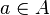# Bijection

A function is bijective if it is both injective and surjective. A bijective function is a bijection (one-to-one correspondence). A function is bijective if and only if every possible image is mapped to by exactly one argument. This equivalent condition is formally expressed as follows.

The functionis bijective iff for all, there is a uniquesuch that f(a) = b.
• A function f : AB is bijective if and only if it is invertible, that is, there is a function g: BA such that g o f = identity function on A and f o g = identity function on B. This function maps each image to its unique preimage.
• The composition of two bijections is again a bijection, but if g o f is a bijection, then it can only be concluded that f is injective and g is surjective. (See the figure at right and the remarks above regarding injections and surjections.)
• The bijections from a set to itself form a group under composition, called the symmetric group.